# Floating point facts for kids

Kids Encyclopedia Facts

Real numbers in binary have to be stored in a special way in a computer. Computers represent numbers as binary integers (whole numbers that are powers of two), so there is no direct way for them to represent non-integer numbers like decimals as there is no radix point. One way computers bypass this problem is floating-point representation, with "floating" referring to how the radix point can move higher or lower when multiplied by an exponent (power).

## Overview

In mathematics and science, very large and very small numbers are often made simpler and multiplied to a power of ten to make them easier to understand. For example, it can be much easier to read 1.2 trillion as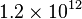$1.2 \times 10^{12}$ than 1,200,000,000,000. This can also be used with negative powers of ten to make small numbers, meaning you can represent 0.000001 as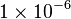$1 \times 10^{-6}$. This process is called scientific notation.

Since computers are limited to integers and binary, this means they cannot easily represent fractional decimal numbers. In order to represent fractional numbers, computers use three sets of binary numbers to make a scientific notation representation. They are: the signed bit, which determines if the number is positive (0) or negative (1); the significand, which is an integer (whole) version of the number; and the exponent, which is the power you multiply the base by.

### Significand

The significand is found by taking your number and moving the radix point until there is no fractional part, making it into an integer. In decimal this is making 1.45 into 145 by moving the point right 2 steps, and in binary this would be making 1101.0111 (13.4375) into 1101 0111 (215) by moving the point right 4 steps; in both cases these numbers aren't related to one another outside using the same digits in a similar ordering.

Similarly to how scientific notation makes the significand as basic as possible, the aim in floating point numbers is to make it an integer so it can be represented in bytes and used in calculations.

### Exponent

The exponent is the number of digits the radix point has moved past: if it moves left then the exponent is negative, but if it moves right then it is positive. As above, making 1.45 into 145 requires you to multiply by 100, so the exponent is 2 as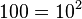$100 = 10^2$. Equally, turning 1101.0111 (13.4375) into 1101 0111 (215) requires you to move the radix point four columns to the right, so the exponent is 4; this can be verified in decimal as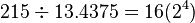$215 \div 13.4375 = 16 (2^4)$.

Since the process is inverted to most cases of scientific notation, as it involves making a faction into an integer rather than turning a large integer into a fraction, exponents are generally negative to move the decimal place to the left; in decimal this would be turning your integer 145 back into the fractional number 1.45 by multiplying it by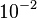$10^{-2}$. Instead of using an signed leftmost bit the exponent is instead biased, giving 32-bit float exponents a range of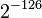$2^{-126}$ to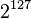$2^{127}$. The output value of the biased exponent can be found by adding 127 to it: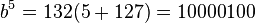$b^5 = 132 (5 + 127) = 1000 0100$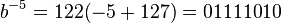$b^{-5} = 122 (-5 + 127) = 0111 1010$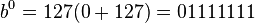$b^0 = 127 (0 + 127) = 0111 1111$

## Example

### Decimal to Bicimal

Let's assume, for example, we want to represent the decimal number 37.40625 to its binary counterpoint: a bicimal (or binary decimal/fraction). First, we need to take our decimal number, which is in powers of 10, and convert it to binary, which is in powers of 2. One way to do this is to subtract the largest power of two possible until you reach zero: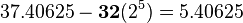$37.40625 - \mathbf{32}(2^5) = 5.40625$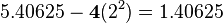$5.40625 - \mathbf{4}(2^2) = 1.40625$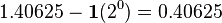$1.40625 - \mathbf{1}(2^0) = 0.40625$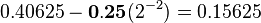$0.40625 - \mathbf{0.25}(2^{-2}) = 0.15625$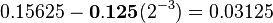$0.15625 - \mathbf{0.125}(2^{-3}) = 0.03125$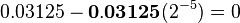$0.03125 - \mathbf{0.03125}(2^{-5}) = 0$

Using the powers of two above, we can represent our decimal number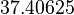$37.40625$ as follows:

Power: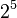$2^5$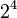$2^4$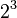$2^3$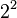$2^2$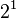$2^1$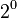$2^0$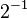$2^{-1}$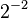$2^{-2}$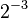$2^{-3}$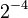$2^{-4}$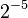$2^{-5}$
Value: 1 0 0 1 0 1 0 1 1 0 1

### Bicimal to Float

We have validated that our decimal number$37.40625$ is represented in binary as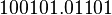$100101.01101$. However, the issue with computers is that they represent numbers as integer powers of two using bits, which makes fractional and negative numbers complicated. In accordance with IEEE-754, the way this is commonly done with a computer is to create a 32-bit floating point number that consists of three parts: the sign, 1 bit to determine if our number is positive or negative; the exponent, 8 bits to represent our exponent and to which we add 127 to avoid signed bytes; and our significand, which is our binary number without a bicimal point spread across 23 bits. Since we need to move our bicimal point 5 places to make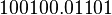$100100.01101$ into the significand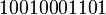$10010001101$ our exponent is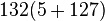$132 (5 + 127)$. By using a 32-bit float we can represent 37.40625 like this:

Type: ±
Exponent Significand
Value: 0 1 0 0 0 0 1 0 0 0 0 1 0 1 0 1 1 0 1 0 0 0 0 0 0 0 0 0 0 0 0 0
Meaning: +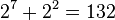$2^7 + 2^2 = 132$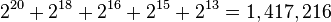$2^{20} + 2^{18} + 2^{16} + 2^{15} + 2^{13} = 1,417,216$

## Images for kidsFloating point Facts for Kids. Kiddle Encyclopedia.